## 3.1 Dynamic Formulas

To provide equations governing relativistic dynamics of matter, we use the Bianchi identities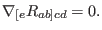(35)

On substituting Eq. (29) into Eq. (35), we get the dynamic formula for the Weyl conformal curvature[3,40,41]: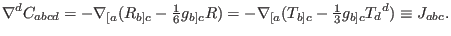(36)

On decomposing Eq. (36) along and orthogonal to a 4-velocity vector, we obtain constraint () and propagation () equations of the Weyl fields in a form analogous to the Maxwell equations[10,42,43,44]: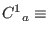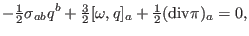(37)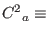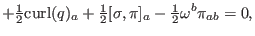(38)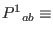(39)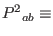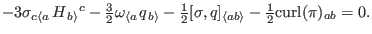(40)

The twice contracted Bianchi identities present the conservation of the total energy momentum tensor, namely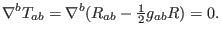(41)

It is split into a timelike and a spacelike momentum constraints: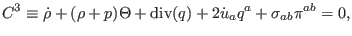(42)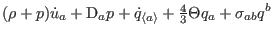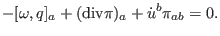(43)

They provide the conservation law of energy-momentum, i. e., how matter determines the geometry, and describe the motion of matter.

Ashkbiz Danehkar
2018-03-26